Select Page

## DBDA- MA

 1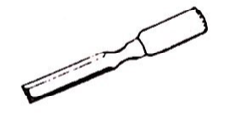On What material would chisel be used? a. Metal b. Wood c. Cement d. Glass e. Plastic 2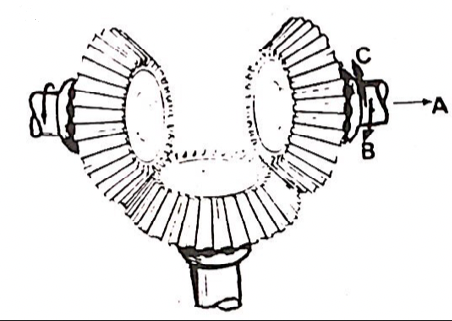If the gear on the left turns in the direction of the arrow, which way does the gear on the right turn? a. A b. B c. C d. Alternately B and C e. The right gear does not turn 3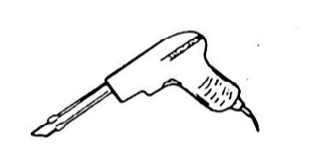What is this tool? a. Drill b. Soldering gun c. Welding torch d. Burning tool e. Paint sprayer 4 A device that does the same things as a fuse does is called a(n) a. Circuit breaker b. Resistor c. Relay d. Alternator e. Voltage regulator 5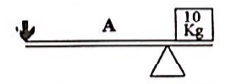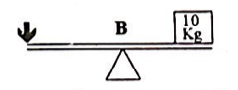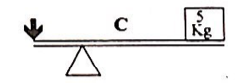In which of the following situations is the least force needed at the arrow, to balance the lever? a. A b. B c. C d. A and C e. B and C 6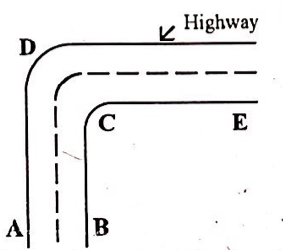Where should the highway be elevated or raised? a. A b. B c. C d. D e. E 7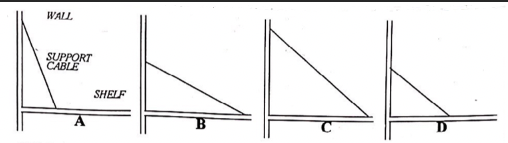Which of the shelves will support the greatest weight? a. A b. B c. C d. D e. They will all support the same weight 8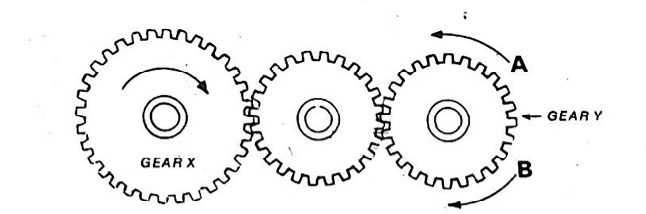If Gear X is turning in the direction of the arrow, in what direction and how fast is Gear Y turning? a. In direction A and the same speed as Gear X b. In direction A and faster than Gear X c. In direction B and slower than Gear X d. In direction B and the same speed as Gear X e. In direction B and faster than Gear X 9 Powdered graphite is useful as a(n) a. Adhesive b. Thickening agent c. Fuel d. Lubricant e. Conducting agent 10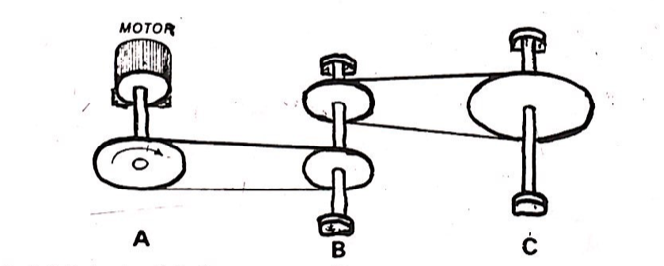Which shaft is turning fastest? a. A b. B c. C d. A and C e. They will all move at the same speed 11 In which of the following lists are the four metals arranged from hardest to softest ? a. Steel, copper, iron, lead b. Steel, iron, lead, copper c. Iron, steel, copper, lead d. Iron, steel, lead, copper e. Steel, iron, copper, lead 12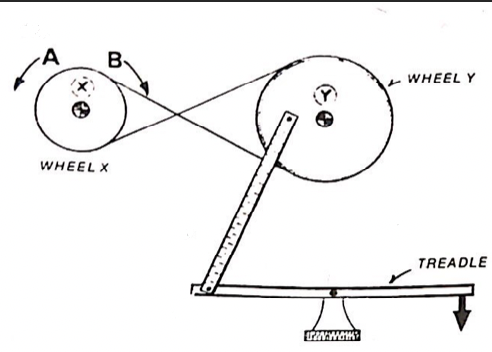In what direction and how fast is wheel X turning as the Treadle moves in the direction of the arrow? a. Direction A and faster than wheel Y b. Direction A and slower than wheel Y c. Direction B and faster than wheel Y d. Direction B and slower than wheel Y e. Direction A and the same speed as wheel Y 13 A basic part of many thermostats is a. A knife switch b. A bimetallic strip c. A spring-activated relay d. An electromagnetic relay e. Photo-sensitive activating device 14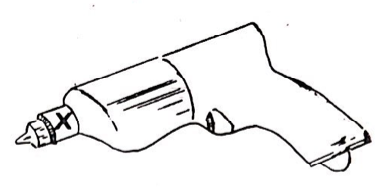What do we call the part of this tool that is marked with an X? a. Bit b. Housing c. Chuck d. Reduction gear e. Key 15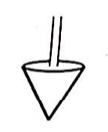This tool is used by a mason for: a. Making holes b. Measuring thickness of walls c. Cementing bricks d. Examining the straightness of walls e. Writing on the walls 16 An automobile carburettor a. Provides a coupling between front and rear wheels b. Changes direct electrical current to alternating current c. Allows the rear wheels to turn at a greater speed d. Converts the up and down motion of the pistons e. Allows the mixture of air and petrol 17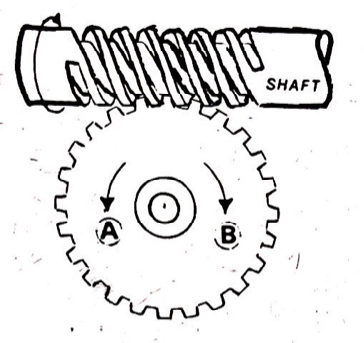The Shaft in the picture cannot move from one side to other, but it can turn. As the shaft is turned in the direction shown by the arrow, which way will the gear turn? a. A b. B c. Alternatively A and B d. First A, then B e. The gear will not turn 18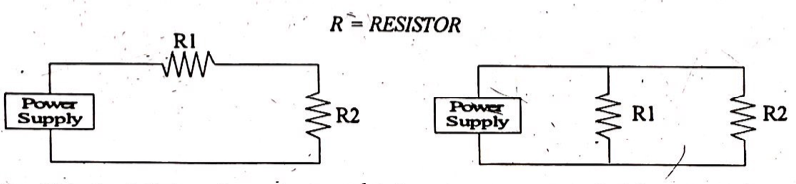In which circuit is the voltage always the same across each resistor, regardless of the values of R1 and R2? a. A b. B c. Both A and B d. Neither A nor B e. It depends on the resistance 19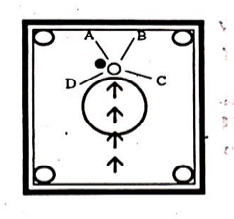After hitting the black coin, which way is the striker likely to go on the carom-board? a. A b. B c. C d. D e. It will rebound 20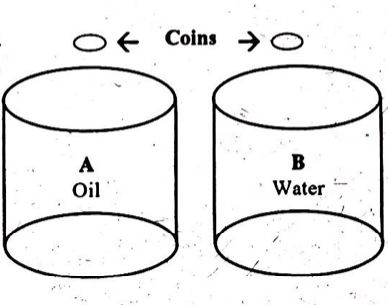If same kind of coins are dropped in these two beakers, which coin will drown faster? a. A b. B c. Both will take equal time d. Coin in beaker A will float e. Coin in beaker B will float 21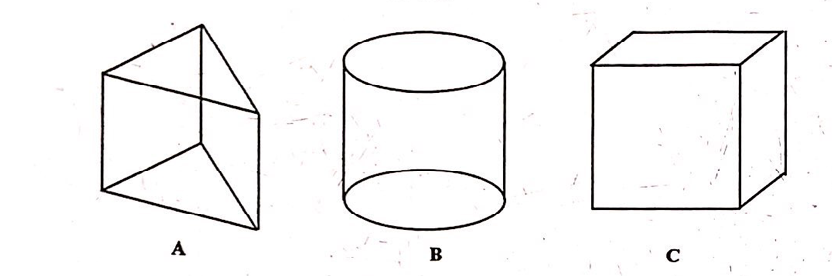Which of the wooden boxes is the heaviest? a. A b. B c. C d. All are equal in weight e. B and C are the heaviest 22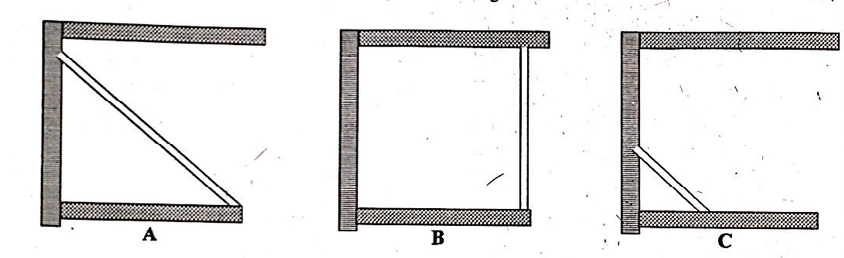Which of these shelves will hold the maximum weight? a. A b. B c. C d. All will hold equal weight e. A and B will hold same weight 23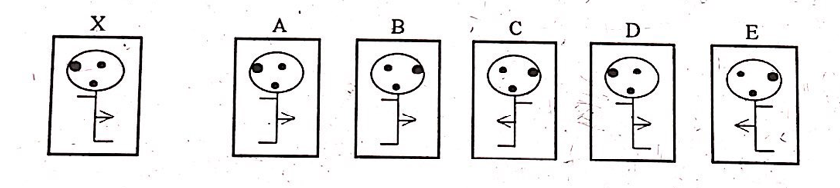How will figure X will look in the mirror? Choose from the figures given below: a. A b. B c. C d. D e. E 24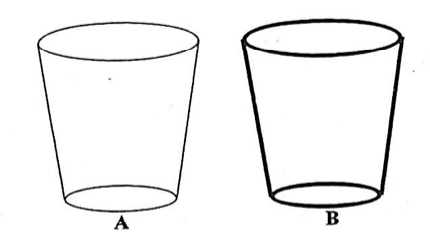Which of these glass will break easily when poured with very hot liquid? a. A b. B c. Both will break at the same time d. Both can retain the hot liquid e. Hot liquid cannot be poured in A 25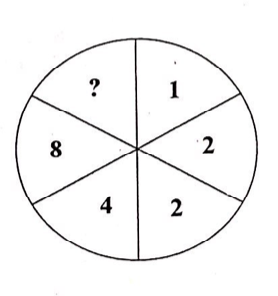Find out the missing number? a. 17 b. 12 c. 32 d. 8 e. 40